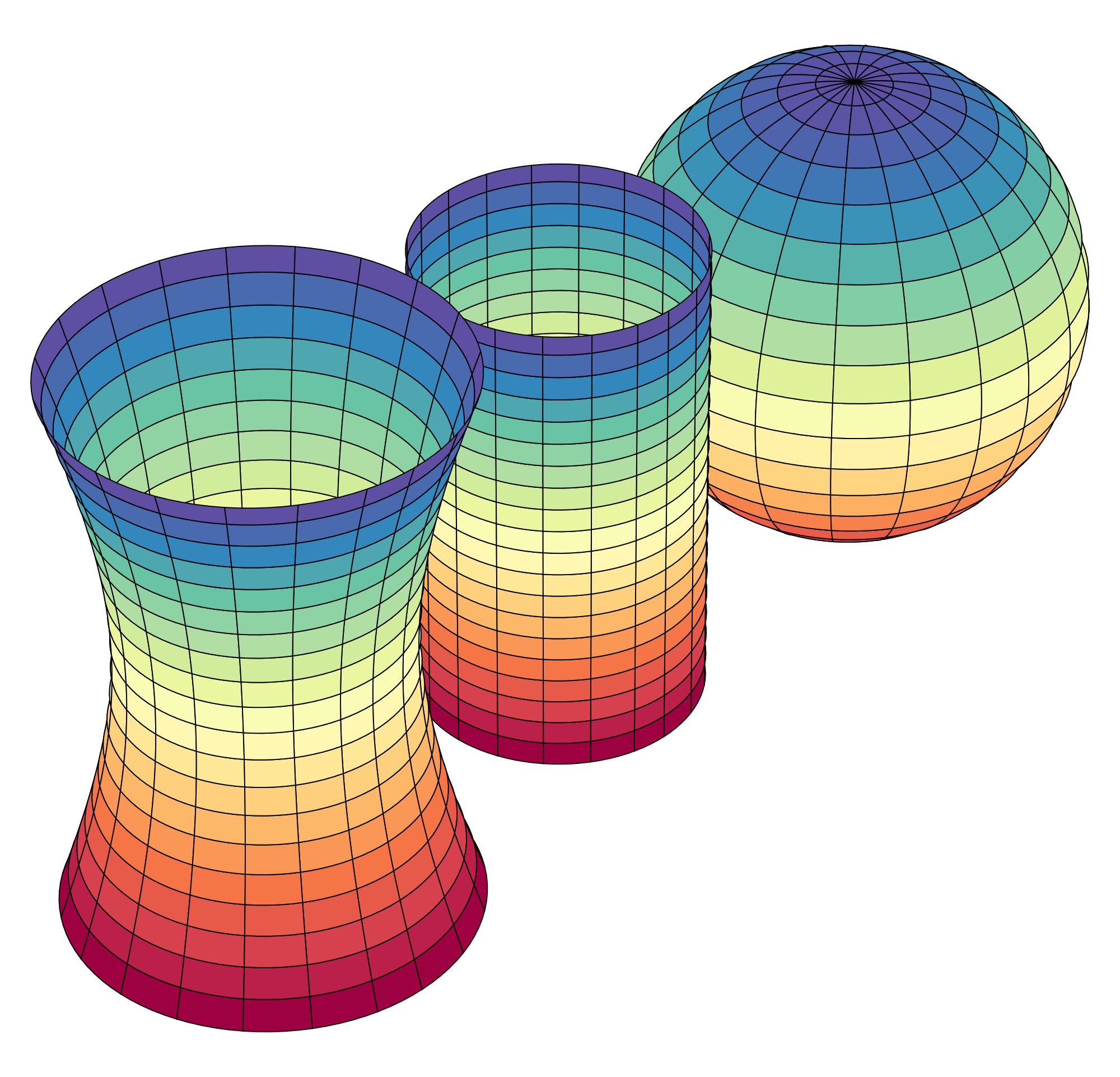# Calculus-Based Statistics - Probability Theory - Distance Calculus Enroll Now, Start Today, Finish Quick - Calculus Academic Credits

If you are shopping around for an Applied Calculus = Survey of Calculus course that you can start immediately, and finish quickly (as quickly as your academic skills allow), then Distance Calculus @ Roger Williams University may be the right program for you.

####Upper Division Math Courses

Our Survey of Calculus = Applied Calculus course is not a "canned" multiple choice course like those offered at many other schools and MOOCs (which usually do not offer the academic credits on academic transcript that you need). Applied Calculus has a wonderful curriculum, providing an excellent introductory study of Differential and Integral Calculus without the rigor (and trigonometry) found in the engineering-level Calculus I course.

Here is a video about earning real academic credits from Distance Calculus @ Roger Williams University:

## Applied Calculus vs Calculus I

Calculus-Based Statistics course can best be described as a "first course in the study of Statistics and Probablity Theory using Calculus".

This course has many names, all being equivalent:
• Probablity Theory
• Calculus-Based Statistics
• Second Course in Statistics for STEM

Our Probability Theory course differs from a classroom/textbook-based course in that we employ Mastery Learning so that you complete all assignments at 100% to assure competancy, as well as our curriculum shifting the course to a laboratory-style course, where theorem/lemma/proof type exposition is replaced by running experiments in Mathematica as you would in a science laboratory to empirically deduce the concepts and behaviors of Probability Theory, both solvable (classically) via hand-based techiques, as well as studying Probability Theory that can only be solved and investigated graphically and numerically using a computer. The Probability Theory curriculum is highly visual and based upon observations of experiments run in Mathematica or LiveMath.

At Distance Calculus, we call our "Calculus-Based Statistics" course as Probability Theory - DMAT 311 - 3 credits.

Below are some links for further information about the Calculus-Based Statistics course via Distance Calculus @ Roger Williams University.

## Distance Calculus - Student ReviewsDate Posted: Jan 12, 2020
Review by: Brian Finley
Courses Completed: Calculus II
Review: I took Calculus II through Distance Calculus and can't recommend it enough. Being able to take the course at my own pace while I was working full time was tremendously helpful, especially since I hadn't taken a math course for 5 years prior. The instruction was excellent and the software they used to teach the course was intuitive and facilitated the learning process very well. This calc II class enabled me to take multivariable calc, linear algebra, and real analysis at Harvard University's extension school, which ultimately qualified me for the economics PhD program that I will graduate from next year. 8 years on, I'm still grateful to Professor Curtis and Distance Calculus.Date Posted: Jun 6, 2020
Review by: Douglas Z.
Courses Completed: Multivariable Calculus, Differential Equations, Linear Algebra, Probability Theory
Review: I loved these courses. So in depth and comprehensive. The mix of software and math curriculum was tremendously helpful to my future studies and career in engineering. I highly recommend these courses if you are bored of textbook courses.
Transferred Credits to: University of Massachusetts, AmherstDate Posted: Jan 8, 2021
Review by: Cristian Mojica
Student Email: comojica@ucdavis.edu
Courses Completed: Probability Theory